# Overview

To facilitate rapid prototyping, the Dune Python bindings provide Python classes for all the core interfaces of Dune. This makes it easy to develop algorithms directly in Python which go beyond what is available through the Dune-Fem discretization module. This is especially of interest for pre- and postprocessing part of the overall simulation package. While most interface methods from the Dune-Fem package are high level, i.e., computationally expensive, many of the core Dune interface methods are not, i.e., the methods for computing the geometric representation of an element or the methods required to iterate over a grid. Consequently, depending on the requirements of the developed code parts, using the Dune core interfaces through Python could incur a too high hit on the performance of the code. Since the exported Python interfaces are very close to the original Dune C++ interfaces, transferring a Python prototype of the algorithm to C++ is mostly straightforward. A just in time compilation utility available in Dune-Python makes it then very easy to use these C++ algorithms from within Python.

In the following we briefly introduce the most important parts of the Dune core interface

# Dense Vectors and the Geometry Classes

A quick survey of Dune-Common and Dune-Geometry The core module Dune-Common provides some classes for dense linear algebra. The FieldVector and FieldMatrix classes are heavily used in the grid geometry realizations. The conceptional basis for these geometries is provided by Dune-Geometry, providing, for example, reference elements and quadrature rules.

:

import time, numpy, sys
import matplotlib.pyplot as pyplot
import dune.fem

from dune.common import FieldVector
x = FieldVector([0.25,0.25,0.25])

import dune.geometry
geometryType = dune.geometry.simplex(2)
referenceElement = dune.geometry.referenceElement(geometryType)
print("\t".join(str(c) for c in referenceElement.corners))

print(p.position, p.weight)

(0.000000, 0.000000)    (1.000000, 0.000000)    (0.000000, 1.000000)
(0.333333, 0.333333) -0.28125
(0.600000, 0.200000) 0.2604166666666667
(0.200000, 0.600000) 0.2604166666666667
(0.200000, 0.200000) 0.2604166666666667


We can also obtain all weights and points stored in numpy arrays which can be useful to vectorize the computation of quadratures:

:

points,weights = dune.geometry.quadratureRule(geometryType, 3).get()

quadrature points: [[0.33333333 0.6        0.2        0.2       ]
[0.33333333 0.2        0.6        0.2       ]]
quadrature weights: [-0.28125     0.26041667  0.26041667  0.26041667]


# Grid Construction and Basic Interface

We now move on to the Dune-Grid module. First let us discuss different possibilities of constructing a grid.

:

from dune.grid import cartesianDomain, yaspGrid
domain = cartesianDomain([0, 0], [1, 0.25], [15, 4])
yaspView = yaspGrid(domain)

yaspView.plot()
yaspView.hierarchicalGrid.globalRefine()
yaspView.plot()Let’s visualize the grid and then globally refine it once

:

from dune.alugrid import aluConformGrid
aluView = aluConformGrid(domain)
aluView.plot()
aluView.hierarchicalGrid.globalRefine()
aluView.plot()## An unstructured grid

can be constructed by providing a dictionary containing vertex coordinate and element connectivity

:

vertices = [(0,0), (1,0), (1,0.6), (0,0.6), (-1,0.6), (-1,0), (-1,-0.6), (0,-0.6)]
triangles = [(2,0,1), (0,2,3), (4,0,3), (0,4,5), (6,0,5), (0,6,7)]
aluView = aluConformGrid({"vertices": vertices, "simplices": triangles})
aluView.plot(figsize=(5,5))
aluView.hierarchicalGrid.globalRefine(2)
aluView.plot(figsize=(5,5))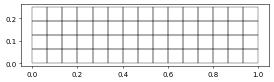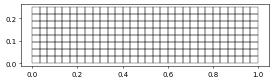An unstructured grid can also be constructed from a file. Currently gmsh and DGF are supported.

In addition to global refinement we can also pre process the grid by marking a subset of elements for local refinement.

:

from dune.grid import Marker
aluView.plot(figsize=(5,5))
for i in range(1,4):
def mark(e):
x = e.geometry.center
return Marker.refine if x.two_norm < 0.64**i else Marker.keep
aluView.plot(figsize=(5,5))

from dune.alugrid import aluSimplexGrid
vertices = aluView.coordinates()
triangles = [aluView.indexSet.subIndices(e, 2) for e in aluView.elements]
aluView = aluSimplexGrid({"vertices": vertices, "simplices": triangles})We next discuss how to retrieve basic information from a constructed grid and iterate over its entities (i.e., elements, faces, edges, vertices, etc.).

:

vertices = [(0,0), (1,0), (1,1), (0,1)]
triangles = [(2,0,1), (0,2,3)]
unitSquare = aluSimplexGrid({"vertices": vertices, "simplices": triangles})
print(unitSquare.size(0),"elements and",unitSquare.size(2),"vertices")

for codim in range(0, unitSquare.dimension+1):
for entity in unitSquare.entities(codim):
print(", ".join(str(c) for c in entity.geometry.corners))

for edge in unitSquare.edges:
print(", ".join(str(c) for c in edge.geometry.corners))

2 elements and 4 vertices
(1.000000, 1.000000), (1.000000, 0.000000), (0.000000, 0.000000)
(0.000000, 0.000000), (0.000000, 1.000000), (1.000000, 1.000000)
(0.000000, 0.000000), (1.000000, 0.000000)
(0.000000, 0.000000), (1.000000, 1.000000)
(0.000000, 0.000000), (0.000000, 1.000000)
(1.000000, 0.000000), (1.000000, 1.000000)
(1.000000, 1.000000), (0.000000, 1.000000)
(0.000000, 0.000000)
(1.000000, 0.000000)
(1.000000, 1.000000)
(0.000000, 1.000000)
(0.000000, 0.000000), (1.000000, 0.000000)
(0.000000, 0.000000), (1.000000, 1.000000)
(0.000000, 0.000000), (0.000000, 1.000000)
(1.000000, 0.000000), (1.000000, 1.000000)
(1.000000, 1.000000), (0.000000, 1.000000)


In the above we have used the geometry method on the entity which provides the geometric mapping between the reference element of the entity and it’s position in physical space. We have used the corners method to retrieve corners of the entity. Other properties and methods are available to provide volume or the integration element needed to compute quadratures of a grid function over the element - which is discussed in the next section.

# Using grid functions

This is a fundamental concept in any grid based discretization package. These are functions that can be evaluated given an entity in the grid and a local coordinate within the reference element of that entity.

:

@dune.fem.function.gridFunction(aluView, name="cos", order=3)
def f(x):
return numpy.cos(2.*numpy.pi/(0.3+abs(x*x)))

@dune.fem.function.gridFunction(aluView, name="hat0", order=3)
def hat0(element,hatx):
return 1-hatx-hatx

hatx = FieldVector([1./3., 1./3.])
maxValue = max(f(e, hatx) for e in f.gridView.elements)

maxValue = max(f(e.geometry.toGlobal(hatx)) for e in f.gridView.elements)

f.plot()
hat0.plot()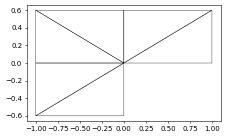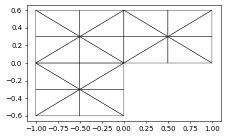We can now use the quadrature rules discuss at the beginning of this chapter to compute the integral over each element of the grid function.

:

from dune.geometry import quadratureRules
integral = 0
for e in aluView.elements:
geo = e.geometry
for qp in rules(e.type):
x,w = qp.position, qp.weight
integral += f(e,x)*w*geo.integrationElement(x)
print("integral of function 'f':",integral)

integral of function 'f': 0.07942992585030194


There are some approach available to improve the efficiency of the computation of integrals since this is an important component of many grid based schemes. Especially, using vectorization can greatly reduce the complexity of the above loop:

:

integral = 0
for e in aluView.elements:
# obtain numpy arrays containing the points and weights and then call
# required functions using these vectors with requiring a loop
points, weights = rules(e.type).get()
ies = e.geometry.integrationElement(points)
values = f(e,points)
integral += numpy.sum(values*ies*weights,axis=-1)
print("integral of function 'f':",integral)

integral of function 'f': 0.07942992585030188


Now a vector valued function

:

# an easy case
dimR = 2
@dune.fem.function.gridFunction(aluView, name="g", order=3)
def g(x):
return [numpy.cos(2.*numpy.pi/(0.3+abs(x*x)))]*dimR
# bit more complex to code
@dune.fem.function.gridFunction(aluView, name="g", order=3)
def g(x):
if type(x) is float:
return [numpy.cos(2.*numpy.pi/(0.3+abs(x*x))),1]
else:
return [numpy.cos(2.*numpy.pi/(0.3+abs(x*x))),*len(x)]
integral = g.dimRange*
for e in aluView.elements:
# obtain numpy arrays containing the points and weights and then call
# required functions using these vectors with requiring a loop
points, weights = rules(e.type).get()
ies = e.geometry.integrationElement(points)
values = g(e,points)
integral += numpy.sum(values*ies*weights,axis=-1)
print("integral of function 'g':",integral)

integral of function 'g': [0.07942993 1.8       ]


# Importing user defined C++ code

To achieve close to native efficiency the Python prototype can be easily reimplemented as a C++ function and the algorithm module used for just in time compilation. This is discussed in a later section based on some examples.

# Attaching Data to the Grid

To attach data to the entities in a grid, each Dune grid has an IndexSet which provides a consecutive, zero starting integer for the set of entities of a given geometry type, i.e., entities sharing the same reference element, like all triangles or all cubes. This can be used to attach data to the these entities stored in random access containers like numpy arrays. To simplify the process further a mapper can be used which is initialized with the data layout, i.e., the number of degrees of freedom that is supposed to be attached to every entity with a given geometry type.

:

mapper = unitSquare.mapper([2, 0, 3])

layout = {dune.geometry.quadrilateral: 4, dune.geometry.triangle: 1}
mapper = unitSquare.mapper(layout)

def interpolate(grid):
mapper = grid.mapper({dune.geometry.vertex: 1})
data = numpy.zeros(mapper.size)
for v in grid.vertices:
data[mapper.index(v)] = f(v.geometry.center)
return mapper, data

mapper, data = interpolate(aluView)
@dune.fem.function.gridFunction(aluView, name="p12d", order=1)
def p12dEvaluate(e, x):
bary = 1-x-x, x, x
idx = mapper.subIndices(e, 2)
return sum(b * data[i] for b, i in zip(bary, idx))

@dune.fem.function.gridFunction(aluView, name="error", order=3)
def error(e, x):
return abs(p12dEvaluate(e, x)-f(e, x))
hatx = FieldVector([1./3., 1./3.])
print(max(error(e, hatx) for e in aluView.elements))

1.6026566867981322


# Output of Grid and Visualization of Data

We already used matplotlib to plot the grid and grid functions. For more flexible plotting Dune relies on vtk and Dune-Python makes it easy to write suitable files.

:

p12dEvaluate.plot(figsize=(9,9), gridLines=None)
p12dEvaluate.plot(figsize=(9,9), gridLines='black',
xlim=[0,0.4], ylim=[0,0.4])
f.plot(level=2, figsize=(9,9), gridLines=None)

pd = {"exact": f, "discrete": p12dEvaluate, "error": error}
aluView.writeVTK("interpolation", pointdata=pd)

aluView.writeVTK("interpolation_subsampled", subsampling=2, pointdata=pd)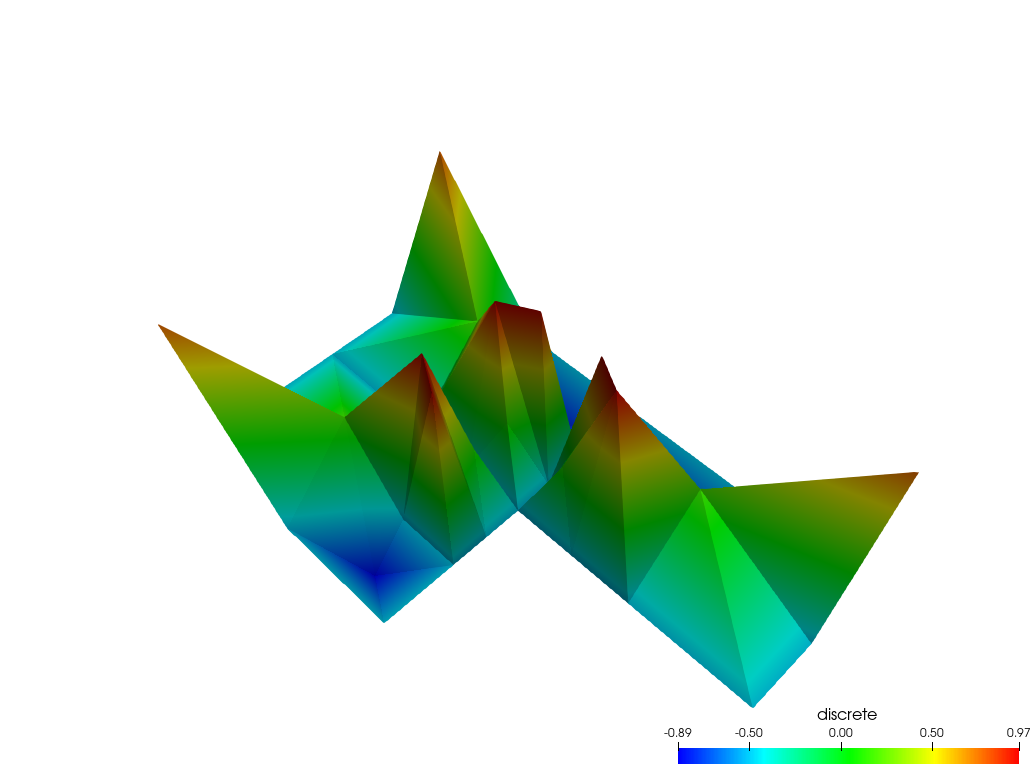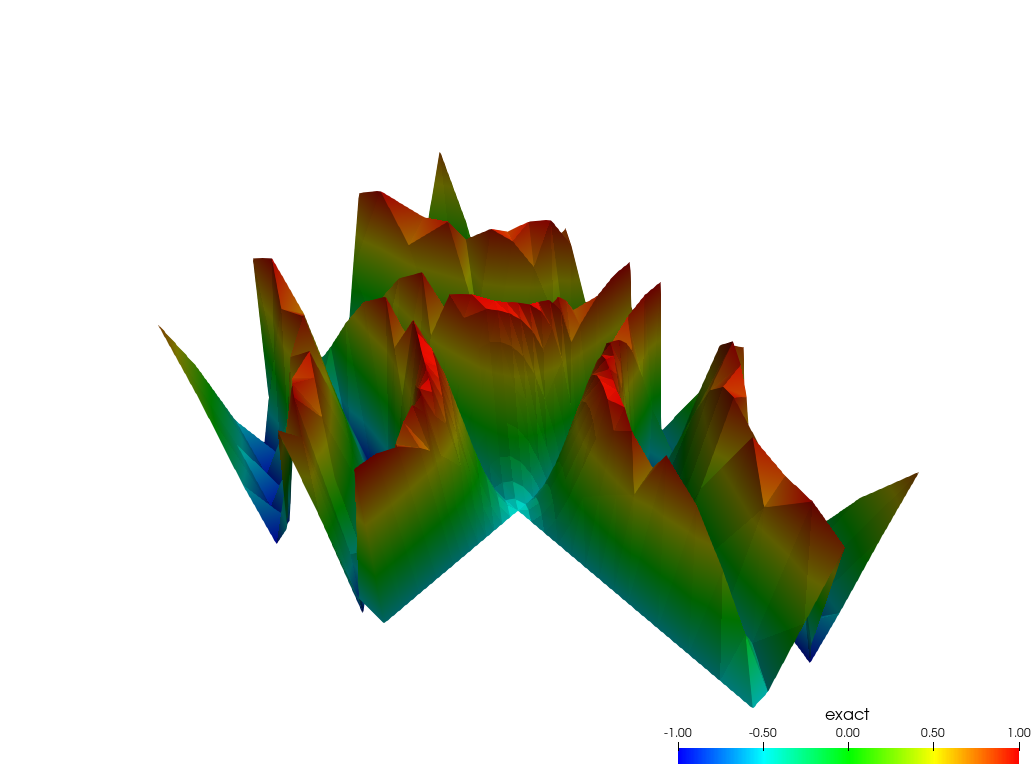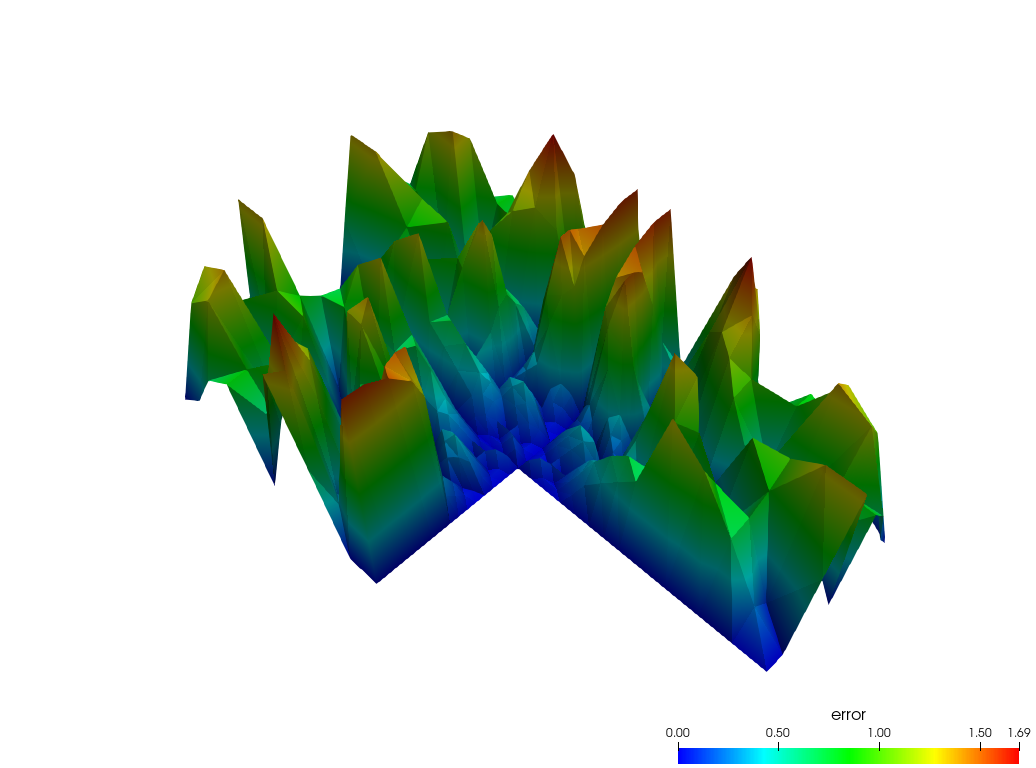Mayavi can also be used to plot grid function in Python. This approach relies on methods to extract numpy representations of the grid data structure and values of a given grid function.

:

level = 3
triangulation = f.gridView.triangulation(level)
z = f.pointData(level)[:,0]
try:
from mayavi import mlab
from mayavi.tools.notebook import display
mlab.init_notebook("png")
mlab.figure(bgcolor = (1,1,1))
s = mlab.triangular_mesh(triangulation.x, triangulation.y, z*0.5,
triangulation.triangles)
display( s )
# mlab.savefig("mayavi.png", size=(400,300))
mlab.close(all=True)
except ImportError:

mayavi module not found so not rendering plot - ignored

This page was generated from the notebook dune-corepy_nb.ipynb and is part of the tutorial for the dune-fem python bindings